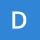Home
IT Knowledge
Inspiration
Languages
EN

# MySQL - Select multiple values

0 points
Created by:Dharman
278

In this article, we would like to show you how to select multiple values in MySQL.

Quick solution:

``````SELECT *
FROM `table_name`
WHERE `column_name` = value_1 OR `column_name` = value_2;``````

or

``````SELECT *
FROM `table_name`
WHERE `column_name` IN(value_1, value_2);``````

## Practical example

To show how to select multiple values, we will use the following `users` table:

Note:

At the end of this article you can find database preparation SQL queries.

### Example 1 - with `OR` condition

In this example, we will select rows from `users` table where `id = 2` or `4` using `OR` condition.

Query:

``````SELECT *
FROM `users`
WHERE `id` = 3 OR `id` = 5;``````

Result:

### Example 2 - with `IN` function

In this example, we will select rows from `users` table where `id = 2` or `4` using `IN` function.

Query:

``````SELECT *
FROM `users`
WHERE `id` IN(2,4);``````

Result:

## Database preparation

`create_tables.sql` file:

``````CREATE TABLE `users` (
`id` INT(10) UNSIGNED NOT NULL AUTO_INCREMENT,
`name` VARCHAR(50) NOT NULL,
`surname` VARCHAR(50) NOT NULL,
PRIMARY KEY (`id`)
);``````

`insert_data.sql` file:

``````INSERT INTO `users`
( `name`, `surname`)
VALUES
('John', 'Stewart'),
('Chris', 'Brown'),
('Kate', 'Lewis'),
('Ailisa', 'Gomez'),
('Gwendolyn', 'James'),
('Simon', 'Collins'),
('Taylor', 'Martin'),
('Andrew', 'Thompson');``````